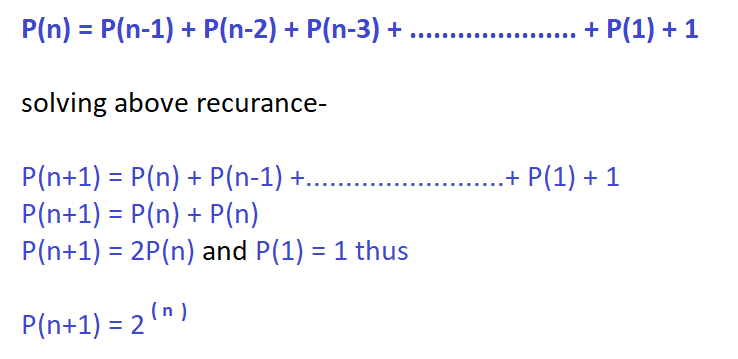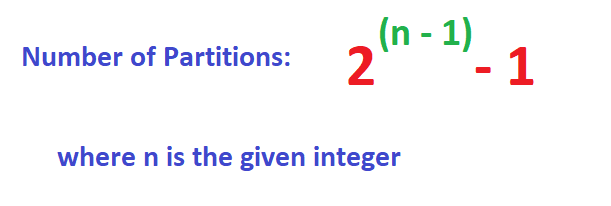×

Search anything:

# Number of non unique Partitions of an Integer

#### Algorithms Dynamic Programming (DP)The problem is to find the number of partitions of a given integer n. Number of partition of an integer is the number of ways the integer can be expressed as a sum of two or more positive integers.

Let us understand the problem using some examples:

``````Input: 3
Output: 3
The partitions will be {1+1+1}, {1+2}, {2+1}.
Input: 4
Output: 7
The partitions will be {1+1+1+1}, {1+1+2}, {1+2+1}, {1+3}, {2+1+1}, {2+2}, {3+1}.
``````

The above problem is similar to coin exchange problem.To simplify the problem we will consider only positive integers.

To solve this problem, we will explore three techniques:

• Brute force/ naive approach: O(N^N)
• Dynamic programming approach: O(N^2)
• Optimal approach: O(log N)

## Naive Approach

A simple approach is to generate all possible combinations recursively and increase the counter after a combination is generated and print the partitions. Thus we will make n-1 recursive calls every time as for each integer n we will fix one partition value( there are n-1 possibilities for 1st partition ) and call for finding remaining partitions. Thus the worst case complexity will be O(n^n). This exponential complexity makes our code very inefficient in terms of time. Here's the pseudo code for the same which returns the number of partitions while printing them.

### Pseudo code

``````print&count_partitions(n, p[], k)
[ n is the given integer, p[] is the partition array and k is the current size of p[] array]
1. Initialize base case where if n=0 then print p[] till k-1 else if n=1 then print p[] till k and then return 1.
2. Iterate i over all values from 1 to n and set p[k]=i
3. Then call recursively for print&count_partitions(n-i, p, k+1) and add the return value of the recursive call to the ans variable.
4. return ans as it determines the number of partitions.
``````

Following is the source code for above pseudo code.

### Source code

``````#include<bits/stdc++.h>
#define ll long long
#define mx 100000
#define f(i,a,b) for(i=a;i<b;i++)

using namespace std;

// All the partitions can be generated using recursive method

ll int number_of_partitions( ll int n, ll int p[], ll int k )
{
if(n==0 || n==1)  //base case: return function if n=1 or n=0 and print the array
{
ll int i=0;
p[k]=n;
f(i,0,k+1)
if(p[i]>0)
cout<<p[i]<<" ";
cout<<endl;
return 1;
}
else {
ll int i,ans=0;
f(i,1,n+1)
{
p[k]=i;
ans+=number_of_partitions(n-i,p,k+1); //recursive calls for similar subproblems
}
return ans;
}
}

int main()
{
ll int t,i,j,n,m,ans=0,c=0;
cout<<"Enter no. of testcases: ";
cin>>t;
while(t--)
{
cout<<"Enter the number(positive integer): ";
cin>>n;
ll int p[n+1];
//remember we dont need to count the number itself in the partitioning at the last
cout<<"Total number of partitions: "<<number_of_partitions(n,p,0)-1<<endl;
}
return 0;
}
``````

### Complexity of Brute force approach

• Time complexity: O(n^n)
• space complexity: O(n)

## Optimal (Dynamic Programming) Approach

While going through the naive approach we realized that we are calculating the answer for smaller subproblems again and again. Thus we will use the Dynamic approach which uses memoization to store the results of smaller subproblems so that they don't need to be evaluated more than once.
The basic idea is that we can express the integer as sum of two other integers which again can be expressed as two smaller integers. We continue dividing and calculating answer until we reach the base case where n=0, n=1 OR we already know the answer for the subproblem which is stored in dp array.
Proceeding to how to divide the problem into subproblems we could always choose a number as the first partition on which our further partitioning depends. Thus we will fix the first partition and recur for possible partitions with the fixed partition as their 1st partition. Thus it would look like-

``````P(5) = 1 + P(4) | 2 + P(3) | 3 + P(2) | 4 + P(1) | 5 + P(0)
Then again, P(4) = 1 + P(3) | 2 + P(2) | 3 + P(1) | 4 + P(0)
In the same way P(3), P(2) can be expressed.
Note: P(1) and P(0) cannot be expressed further. You may notice that n + P(0) doesnt makes sense as 0 does not represent a positive integer. But we realize that this partition is also necessary to gain all the counts.
``````

Following is the implementation of the above dynamic approach. Here we have only calculated number of partitions. If we want to print the partitions using dynamic approach it will take O(2^n) complexity.

### Source code

``````#include<bits/stdc++.h>
#define ll long long
#define mx 100000
#define f(i,a,b) for(i=a;i<b;i++)

using namespace std;

// The total number of partitions can be evaluated using two dynamic programming approaches :
// 1.Recursive approach
// 2.Iterative approach
// Here is the recursive approach as to get the basic idea of how recursion works

ll int dp[mx]; // dp array for memoization
ll int number_of_partitions( ll int n )
{
if( dp[n]!=-1 )  //base case: return function if dp[n] already calculated
return dp[n];
else {
ll int i,ans=0;
f(i,1,n+1)
{
ans+=number_of_partitions(n-i); //recursive calls for similar subproblems
}
return dp[n]=ans;
}
}

int main()
{
ll int t,i,j,n,m,ans=0,c=0;
cout<<"Enter no. of testcases: ";
cin>>t;
while(t--)
{
cout<<"Enter the number(positive integer): ";
cin>>n;
dp=dp=1;
f(i,2,n+1)
dp[i]=-1;
//remember we dont need to count the number itself in the partitioning at the last
cout<<"Total number of partitions: "<<number_of_partitions(n)-1<<endl;
}
return 0;
}
``````

### Complexity

• Time complexity: O(n^2)
• Space complexity: O(n)

## More optimal approach

After reading up to this some of you (who may already be aware of the idea of basic dynamic programming or who may have solved the coin exchange problem) might be thinking that what is the difference here, what is the learning here in this problem. So what if I told you that this can be solved with much lesser complexity without even using O(n) auxiliary space. We will use mathematical approach to count the combinations possible.

Lets Consider the number of compositions of the positive integer in which order does matter as P(n). For example if n=5 then P(n) = 15.Thus we can see that if we fix one partition as k then the remaining composition is number of partitions of n-k i.e. P(n-k). ThereforeWe would use -1 for excluding the number itself as we need partitions of 2 or more elements. Also we already know we can calculate pow(2,n-1) easily using Modular Exponentiation in O(logn) time for smaller n.### Pseudo code

``````[ no_of_partitions(n) = Power(2,n-1)-1 ]
Power(x,n)
1. Initialize result as 1.
2. Now repeat steps 3 to 5 till n reduces to 0
3. If n is odd multiply x to the result
4. Divide n by 2
5. Update x as x*x
6. return result
``````

Following is the source code for the above pseudo code.

### Source code

``````#include<bits/stdc++.h>
#define mx 100000
#define ll long long
#define f(i,a,b) for(i=a;i<b;i++)

using namespace std;

//power function for fast exponentiation
ll int power(ll int x, ll int n)
{
ll int res=1;
while(n>0)
{
if(n&1)
res*=x;
n=n>>1;
x=x*x;
}
return res;
}

int main()
{
ll int t,n;
cout<<"Enter the no of test cases: ";
cin>>t;
while(t--)
{
cout<<"Enter the number(positive integer): ";
cin>>n;
cout<<"No of partitions: "<<power(2,n-1)-1<<endl;
}
return 0;
}
``````

### Complexity

Time Complexity: O(log N) using fast exponentiation